## 神经网络预训练方案

• RBM：Hinton et al., 2006; Hinton and Salakhutdinov, 2006; Lee et al., 2008
• auto-encoder：Bengio et al., 2007; Ranzato et al., 2007
• semi-supervised embedding：Weston et al.,2008
• kernel PCA：Cho and Saul, 2010

RBM 和 自编码 模型函数形式很像，但是训练和解释都不同。一个很大不同是，确定性的自编码可以用实数值作为隐层的表达，

autoencoder 的重构误差可以看做 RBM 的 log-likelihood gradient 一种近似。
RBM 中的 Contrastive Divergence 更新。

## 好的特征表达： 交互信息 到 自编码

A good representation is one that will yield a better performing classifier

### 独立成分分析（ICA）：Bell and Sejnowski (1995)

$$\arg \max_{\theta} \mathbb{I}(X; Y) = \arg \max_{\theta} - \mathbb{H}(X|Y) \\ = \arg \max_{\theta} \mathbb{E}_{q(X, Y)} [\log q(X|Y)]$$

$$\mathbb{E}_{q(X, Y)} [\log p(X|Y)] \le - \mathbb{H}(X|Y)$$

$$\max_{\theta, \theta'} \mathbb{E}_{q(X, Y; \theta)} [\log p(X|Y; \theta')]$$

$$\max_{\theta, \theta'} \mathbb{E}_{q^0(X)} [\log p(X|Y=f_{\theta}(X); \theta')]$$

UFLDL里面的独立成分分析

$$\min || W x ||_1 \\ s. t. WW^T = I$$

$$W = W - \alpha \nabla_W || W x ||_1 \\ W = (WW^T)^{-1/2} W$$

### 传统自编码

$$y = f_{\theta}(x) = s(Wx+b) \\ \theta = \{ W, b \}$$

$$z = g_{\theta'}(y) = s(W' y + b') \\ \theta' = \{ W', b' \}$$

$$L(x, z) = \varpropto - \log p(x | z)$$

1. 对于实数值$x \in \mathbb{R}^d, X|z \sim \mathcal{N}(z, \sigma^2 I$，那么重构误差对应于均方误差$L(x, z) = C(\sigma^2)||x-z||^2$。
2. 对于二进制变量 $x \in \{ 0, 1 \}, X|z \sim \mathcal{B}(z)$，那么重构误差对应于交叉熵损失函数。

autoenoder 的训练最小化重构误差，即优化下列问题：

$$\arg \min_{\theta, \theta'} \mathbb{E}_{q^0(X)} L(X, Z=g_{\theta'}(f_{\theta}(X)))$$

$$\arg \max_{\theta, \theta'} \mathbb{E}_{q^0(X)} \log(p(X| Y=f_{\theta}(X); \theta'))$$

training an autoencoder to minimize reconstruction error amounts
to maximizing a lower bound on the mutual information between input X and learnt representation Y

The use of “tied weights” can also change the solution: forcing encoder and decoder matrices to
be symmetric and thus have the same scale can make it harder for the encoder to stay in the linear
regime of its nonlinearity without paying a high price in reconstruction error.

## Variational autoencoders 论文导读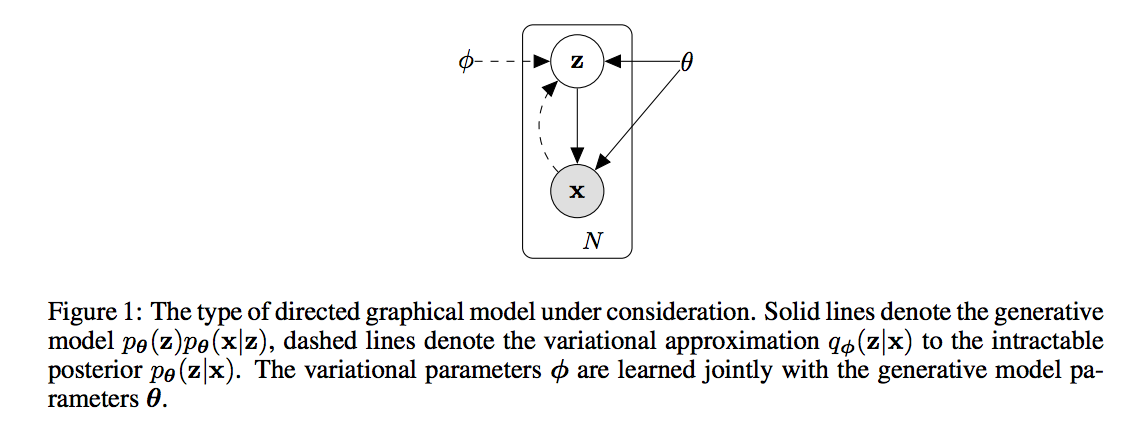N个iid样本$x^{(i)}$，连续或离散值。假定这些数据从一些随机过程产生！

1. 从先验分布$p_{\theta^* }(z)$产生隐变量$z$。
2. 从条件概率$p_{\theta^* }(x|z)$产生$x$。

1. 边际分布$p(x)$需要计算一个积分，对于神经网络等复杂模型，难以求导！EM 算法失效；
2. 大数据集，batch优化没有效率，需要随机梯度之类的优化

1. 有效地近似地实现 ML 和 MAP估计参数$\theta$
2. 给定x，推导z，有效的近似算法
3. 有效的估计x的边际分布

### 变分界 variational bound

$$\log p(x^{(1)}, ..., x^{(N)}) = \sum_i \log p_{\theta} (x^{(i)})$$

$$\log p_{\theta} (x^{(i)}) = D_{KL}(q_{\phi}(z|x^{(i)}) || p_{\theta} (x^{(i)})) \\ + \mathcal{L}(\theta, \phi; x^{(i)}) \\ \mathcal{L}(\theta, \phi; x^{(i)}) = \mathbb{E}_{q_{\phi(z|x)}}[-\log q_{\phi}(z|x) + \log p_{\theta}(x, z)] \\ = - D_{KL}(q_{\phi}(z|x^{(i)}) || p_{\theta}(z)) + \mathbb{E}_{q_{\phi(z|x^{(i)}}} \left[ \log p_{\theta}(x^{(i)} | z) \right]}$$

### The SGVB estimator and AEVB algorithm

$$\mathbb{E}_{q_{\phi}(z|x^{(i)})}[f(z)] \approx \frac{1}{L} \sum_{l=1}^L f(g_{\phi}(\epsilon^{(l)}, x^{(i)})), \\ \epsilon^{(l)} \sim p(\epsilon)$$

mini-batch方法：

$$\mathcal{L}(\theta, \phi; X) \approx \tilde{\mathcal{L}}^M(\theta, \phi; x^M) \\ = \frac{N}{M} \sum_{i=1}^M \tilde{\mathcal{L}}(\theta, \phi; x^{(i)})$$

${X^M}$ 是随机从全部数据集采样的M个数据。

auto-encoder角度：似然函数的第一项相当于正则，第二项是重构误差

## Stacked What-Where Auto-encoders

What: 就是polling后得到的max值，而where是最大值所在的位置信息，用来帮助解卷积器重构！

$$L = L_{NLL} + \lambda_{L2rec} L_{L2rec} + \lambda_{L2M} L_{L2M}$$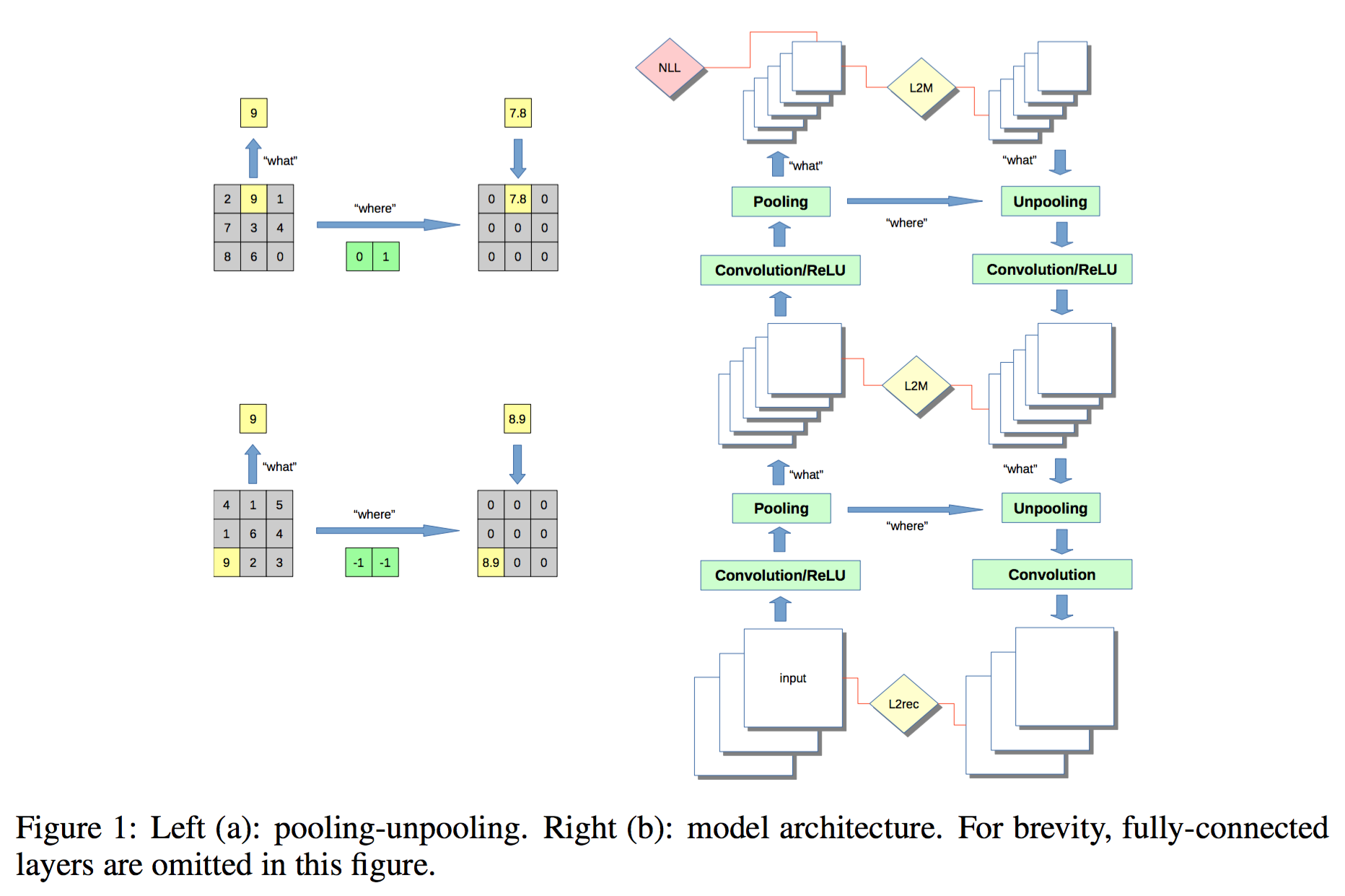### 软最大值max和argmax

Ross Goroshin, Michael Mathieu, and Yann LeCun. Learning to linearize under uncertainty. arXiv preprint arXiv:1506.03011, 2015.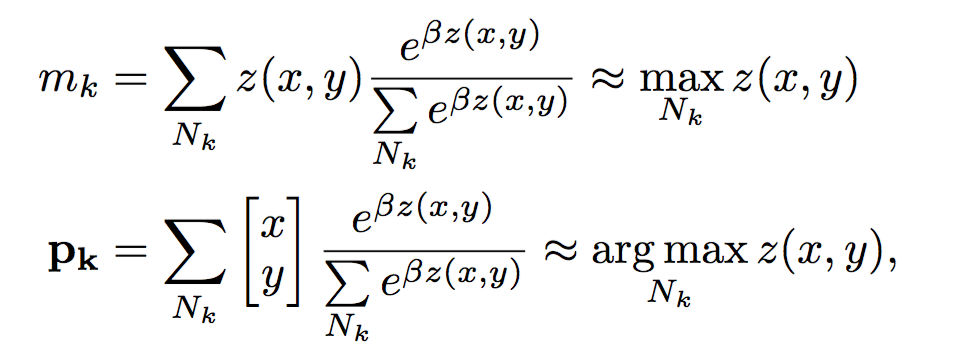### where的重要性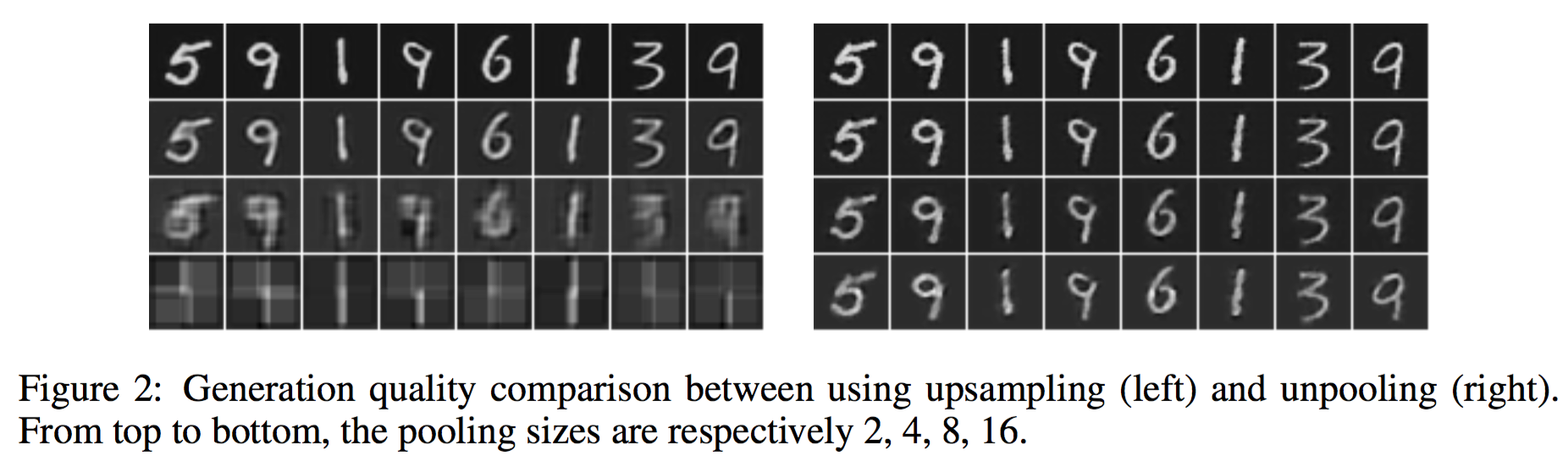what学习到的具有平移不变性！

### 结论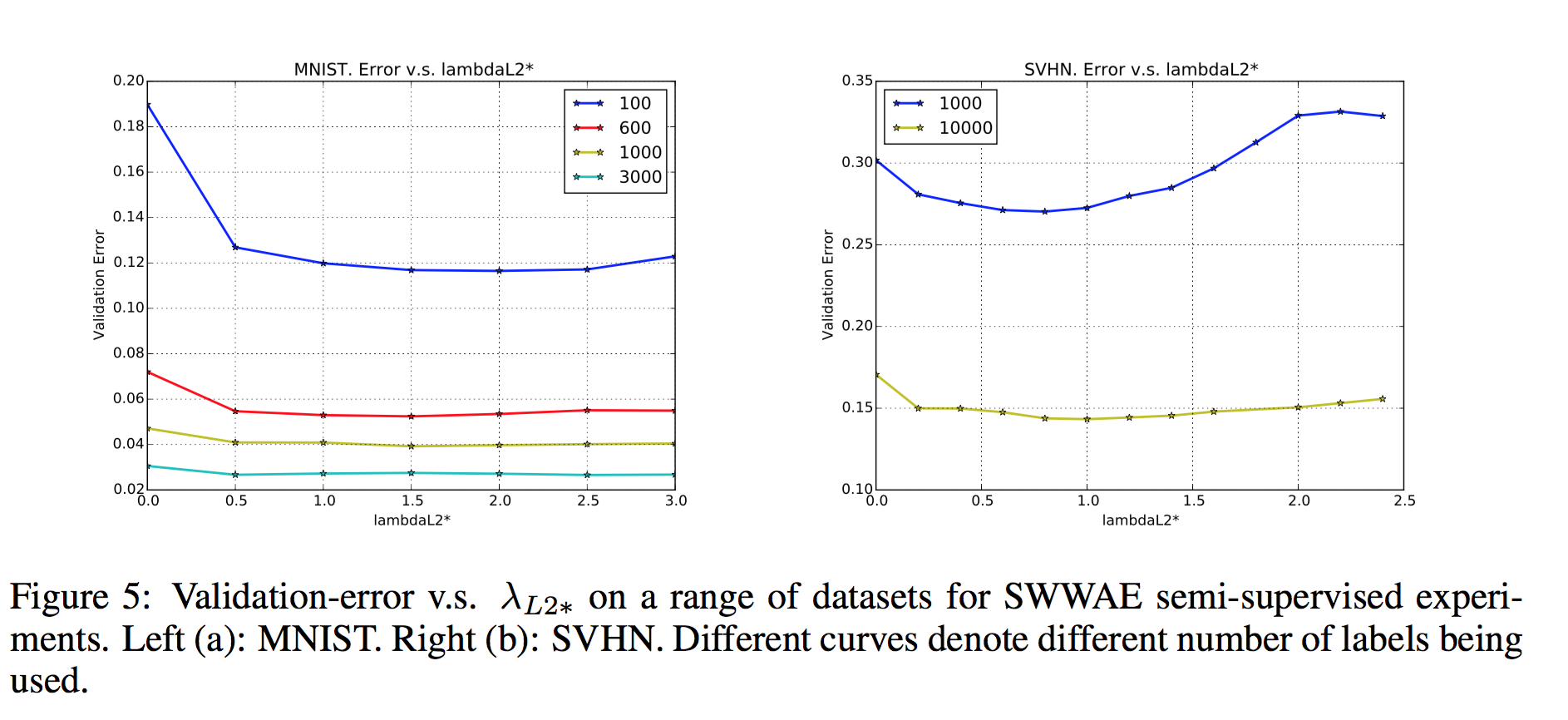## Reference

1. Hinton, G. E. and Salakhutdinov, R. R. Reducing the dimensionality of data with neural networks. Science 2006.
2. 2010, Pascal Vincent, Yoshua Bengio, Stacked Denoising Autoencoders: Learning Useful Representations in a Deep Network with a Local Denoising Criterion.

1. Richard Socher, Jeffrey Pennington, Eric H. Huang, Andrew Y. Ng, and Christopher D. Manning.
Semi-supervised recursive autoencoders for predicting sentiment distributions. In
EMNLP, 2011.
2. Variational autoencoders